# 极限和连续#

——恩格斯

## 变化率和曲线的切线#$y = \frac{1}{2}gt^2$

$y=4.9t^2$

$\frac{\Delta{y}}{\Delta{t}}=\frac{4.9(t_0+h)^2-4.9t_0^2}{h}$
• 如果 $$t_0=1$$ ，则上式为： $$$\frac{\Delta{y}}{\Delta{t}}=\frac{4.9(1+h)^2-4.9(1)^2}{h}=9.8 + 4.9h$$$

$$h$$ 很小——你说多小，比你说的还小，或者说 $$h\to{0}$$ 时，$$\frac{\Delta{y}}{\Delta{t}}\to{9.8}$$

• 如果 $$t_0=2$$ ，则： $$$\frac{\Delta{y}}{\Delta{t}}=\frac{4.9(2+h)^2-4.9(2)^2}{h}=19.6+4.9h$$$

$> \frac{\Delta{y}}{\Delta{x}} = \frac{f(x_2)-f(x_1)}{x_2-x_1}=\frac{f(x_1+h)-f(x_1)}{h} >$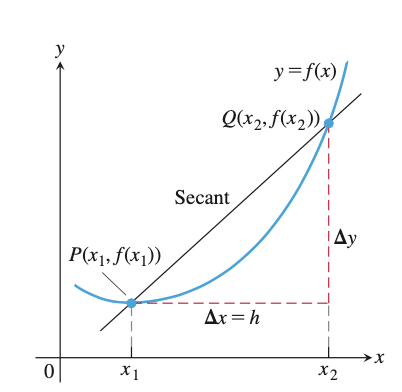## 数列的极限#

$$n$$ 越来越大，以至于无穷大时，$$a_n$$ 便跟着越来越靠近 $$L$$ 。那么我们说，当 $$x\rightarrow\infty$$ 时，$$a_n\rightarrow L$$ 。若以极限式的写法，即为：

$> \lim_{x\rightarrow\infty}a_n=L >$

• 例如：$$a_n=(-1)^n$$ ，数列的取值在 $$1$$$$-1$$ 两个数上更换，并不趋近一个定值；

• 趋近无穷大，即：$$\lim\limits_{x\to\infty}a_n=\infty$$

$$\lim\limits_{n\to\infty}a_n=\alpha,\lim\limits_{n\to\infty}b_n=\beta$$ ，及 $$c\in\mathbb{R}$$ ，则：

• 相加：$$\lim\limits_{n\to\infty}(a_n\pm b_n)=\lim\limits_{n\to\infty}a_n\pm\lim\limits_{n\to\infty}b_n=\alpha\pm\beta$$

• 常倍数：$$\lim\limits_{n\to\infty}c\cdot a_n=c\cdot\lim\limits_{n\to\infty}a_n=c\cdot\alpha$$

• 相乘：$$\lim\limits_{n\to\infty}(a_n\cdot b_n)=\lim\limits_{n\to\infty}a_n\cdot\lim\limits_{n\to\infty}b_n=\alpha\cdot\beta$$

• 相除：$$\lim\limits_{n\to\infty}\frac{a_n}{b_n}=\frac{\lim\limits_{n\to\infty}a_n}{\lim\limits_{n\to\infty}b_n}=\frac{\alpha}{\beta},(\beta\ne0)$$

$> a_n\le b_n\le c_n >$

$> \lim_{n\to\infty}a_n=\lim_{n\to\infty}c_n=L >$

$> \lim_{n\to\infty}b_n=L >$

• 求极限 $$\lim\limits_{n\to\infty}\frac{n!}{n^n}$$

解：

$\frac{n!}{n^n}=\frac{1}{n}\times\frac{2}{n}\times\frac{3}{n}\times\cdots\times\frac{n-1}{n}\times\frac{n}{n}$

显然，展开式的每一项都小于 1，大于 0，故：

$0\le\frac{1}{n}\times\frac{2}{n}\times\frac{3}{n}\times\cdots\times\frac{n-1}{n}\times\frac{n}{n}\le\frac{1}{n}$

由于 $$\lim\limits_{n\to\infty}0=0=\lim\limits_{n\to\infty}\frac{1}{n}$$

由“夹逼定理”得：$$\lim\limits_{n\to\infty}\frac{n!}{n^n}=0$$

• 求极限 $$\lim\limits_{n\to\infty}\frac{\sin(n)}{n}$$

解：

\begin{split} \begin{aligned} &-1\le\sin(n)\le1\Rightarrow-\frac{1}{n}\le\frac{\sin(n)}{n}\le\frac{1}{n} \\&\text{lim}_{n\to\infty}-\frac{1}{n}=0=\text{lim}_{n\to\infty}\frac{1}{n} \\&\text{lim}_{n\to\infty}\frac{\sin(n)}{n}=0 \end{aligned} \end{split}
• 求极限 $$\lim\limits_{n\to\infty}\left(\frac{1}{\sqrt{n^2+1}}+\frac{1}{\sqrt{n^2+2}}+\cdots+\frac{1}{\sqrt{n^2+n}}\right)$$

解：

$\frac{1}{\sqrt{n^2+n}}+\cdots+\frac{1}{\sqrt{n^2+n}}\le\frac{1}{\sqrt{n^2+1}}+\cdots+\frac{1}{\sqrt{n^2+n}}\le\frac{1}{\sqrt{n^2+1}}+\cdots+\frac{1}{\sqrt{n^2+1}}$

因为：

\begin{split} \begin{aligned} &\text{lim}_{n\to\infty}\left(\frac{1}{\sqrt{n^2+n}}+\cdots+\frac{1}{\sqrt{n^2+n}}\right)=\text{lim}_{n\to\infty}\frac{n}{\sqrt{n^2+n}}=1 \\&\text{lim}_{n\to\infty}\left(\frac{1}{\sqrt{n^2+1}}+\cdots+\frac{1}{\sqrt{n^2+1}}\right)=\text{lim}_{n\to\infty}\frac{n}{\sqrt{n^2+1}}=1 \end{aligned} \end{split}

故：

$\text{lim}_{n\to\infty}\left(\frac{1}{\sqrt{n^2+1}}+\frac{1}{\sqrt{n^2+2}}+\cdots+\frac{1}{\sqrt{n^2+n}}\right)=1$

注意：无穷多个无穷小项之和，不一定就是无穷小。

## 函数的极限#

$\lim_{x\to{c}}f(x)=L$

### 定理1：极限运算法则#

$$L,M, c, k$$ 为实数，并且函数 $$f(x)$$$$g(x)$$ 的极限分为别：

$\lim_{x\to{c}}f(x) = L,\quad \lim_{x\to{c}}g(x)=M$

• 加法：$$\lim\limits_{x\to{c}}(f(x)+g(x))=L+M$$

• 减法：$$\lim\limits_{x\to{c}}(f(x)-g(x))=L-M$$

• 数量乘法：$$\lim\limits_{x\to{c}}(k\cdot{f(x)})=k\cdot{L}$$

• 乘法：$$\lim\limits_{x\to{c}}(f(x)\cdot{g(x)})=L\cdot{M}$$

• 商：$$\lim\limits_{x\to{c}}\frac{f(x)}{g(x)}=\frac{L}{M},M\ne{0}$$

• 指数：$$\lim\limits_{x\to{c}}[f(x)]^n=L^n, n是正整数$$

• 开方：$$\lim\limits_{x\to{c}}\sqrt[n]{f(x)}=\sqrt[n]{L}=L^{1/n}$$

### 定理2：多项式的极限#

$\lim_{x\to{c}}P(x)=P(c)=a_nc^n+a_{n-1}c^{n-1}+\cdots+a_0$

### 定理3：多项式商的极限#

$$P(x)$$$$Q(x)$$ 分别是两个多项式，且 $$Q(c)\ne{0}$$ ，则：

$\lim_{x\to{c}}\frac{P(x)}{Q(x)}=\frac{P(c)}{Q(c)}$

### 定理4：三明治定理#

$$x$$ 的区间内，$$g(x)\le{f(x)}\le{h(x)}$$ ，并且常数 $$c$$ 也在此区间内，若：

$\lim_{x\to{c}}g(x)=\lim_{x\to{c}}h(x)=L$

：（1）$$\lim\limits_{x\to0}\sin\theta=0$$ （2）$$\lim\limits_{x\to0}\cos\theta=1$$

（1）在上一节得到了结论：$$-|\theta|\le\sin\theta\le|\theta|$$ ，因为 $$\lim\limits_{\theta\to0}(-|\theta|)=\lim\limits_{\theta\to0}(|\theta|)=0$$ ，根据三明治定理，所以：

$\lim_{\theta\to0}\sin\theta=0$

（2）因为 $$0\le{1-\cos\theta}\le|\theta|$$ ，所以 $$\lim\limits_{\theta\to0}(1-\cos\theta)=0$$ ，则：

\begin{split} \begin{aligned} &\lim_{\theta\to0}(1-(1-\cos\theta))=1-\lim_{\theta\to0}(1-\cos\theta)=1-0 \\&\lim_{\theta\to0}\cos\theta=1 \end{aligned} \end{split}

### 极限定义#

$|f(x)-L|\lt\epsilon$

#### 例题#

$\begin{split} \begin{split}|f(x)+g(x)-(L+M)| &= |(f(x)-L)+(g(x)+M)|\\&\le|f(x)-L|+|g(x)-M| \quad(根据三角不等式\quad |a+b|\le|a|+|b|)\end{split} \end{split}$

$|f(x)-L|\lt\frac{\epsilon}{2}$

$|g(x)-M|\lt\frac{\epsilon}{2}$

$$\delta=\min\{\delta_1,\delta_2\}$$ ，如果 $$0\lt|x-c|\lt\delta$$ ，则 $$|x-c|\lt\delta_1$$ ，故 $$|f(x)-L|\lt\frac{\epsilon}{2}$$ 成立；同样，在此条件下，也有 $$|x-c|\lt\delta_2$$ ，故 $$|g(x)-M|\lt\frac{\epsilon}{2}$$ 成立。

## 左极限和右极限#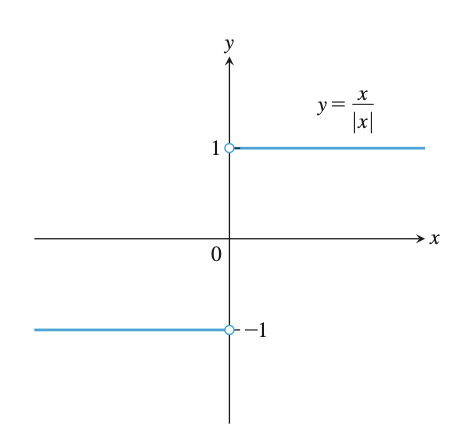• 左极限：$$\lim\limits_{x\to{c}^-}f(x)=M$$

• 右极限：$$\lim\limits_{x\to{c}^+}f(x)=L$$

• 双侧极限：$$\lim\limits_{x\to{c}}f(x)=L\quad\Leftrightarrow\quad \lim\limits_{x\to{c}^-}f(x)=L \quad{and}\quad \lim\limits_{x\to{c}^+}f(x)=L$$

### 证明：$$(\sin\theta)/\theta$$ 的极限#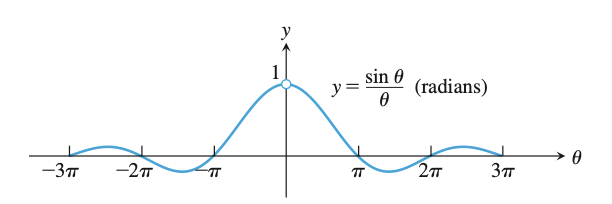θ从双侧趋近0，函数极限都是1

$$\Delta{OAP}的面积\lt扇形OAP的面积\lt\Delta{OAT}的面积$$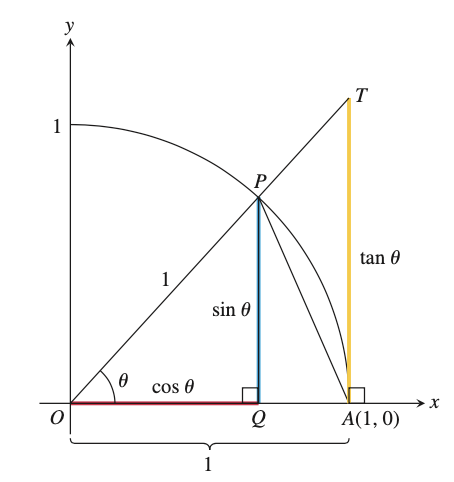$\begin{split} \begin{split}\Delta{OAP}的面积=&S_{\Delta{OAP}}=\frac{1}{2}|OA||PQ|=\frac{1}{2}\sin\theta\\扇形OAP的面积=&S_{\overset{\frown}{OAP}}=\frac{1}{2}r^2\theta=\frac{\theta}{2}\\\Delta{OAT}的面积=&S_{\Delta{OAT}}=\frac{1}{2}|OA||TA|=\frac{1}{2}\tan\theta\end{split} \end{split}$

$\frac{1}{2}\sin\theta\lt\frac{1}{2}\theta\lt\frac{1}{2}\tan\theta$

$1\lt\frac{\theta}{\sin\theta}\lt\frac{1}{\cos\theta}$

$1\gt\frac{\sin\theta}{\theta}\gt\cos\theta$

$\lim_{\theta\to{0}^+}\frac{\sin\theta}{\theta}=1$

$\lim_{\theta\to{0}^-}\frac{\sin\theta}{\theta}=1=\lim_{\theta\to{0}^+}\frac{\sin\theta}{\theta}$

## 连续#

• 如果 $$\lim\limits_{x\to{c}}f(x)=f(c)$$ ，则函数 $$f$$$$c$$ 连续；

• 如果 $$\lim\limits_{x\to{c}^+}f(x)=f(c)$$ ，则函数 $$f$$$$c$$ 右连续；

• 如果 $$\lim\limits_{x\to{c}^-}f(x)=f(c)$$ ，则函数 $$f$$$$c$$ 左连续。

### 连续性检验#

1. $$f(c)$$ 存在（ $$c$$$$f$$ 的定义域内）

2. $$\lim\limits_{x\to{c}}f(x)$$ 存在（当 $$x\to{c}$$$$f$$ 有极限）

3. $$\lim\limits_{x\to{c}}f(x)=f(c)$$ （极限等于函数值 $$f(c)$$

### 连续函数#

• 加法：$$f+g$$

• 减法：$$f-g$$

• 数乘：$$k\cdot{f}$$$$k$$ 是任意一个数

• 乘积：$$f\cdot{g}$$

• 相除：$$\frac{f}{g},g\ne{0}$$

• 幂运算：$$f^n$$$$n$$ 是正整数

• 开方：$$\sqrt[n]{f}$$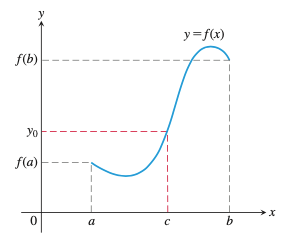### 中值定理#

$f(c)=\mu,\quad a\lt{c}\lt{b}$

$$S$$ 是满足 $$f(x)\lt\mu, a\le{x}\lt{b}$$ 的实数 $$x$$ 的全体集合。

$\because{f(a)}\lt\mu,\quad\therefore a\in S$

$$S$$ 的上确界为 $$c$$ ，如果 $$c\notin S$$ ，则存在收敛于 $$c$$ 的数列 $$\{x_n\}, x_n\in S$$ ，因此 $$f(c)=\lim\limits_{x\to\infty}f(x_n)\le\mu$$ ，从而 $$c\in S$$$$f(c)\le\mu$$ 。这里若假设 $$f(c)\lt\mu$$ ，因为 $$f(x)$$ 是连续函数，所以满足条件 $$|x-c|\lt\delta, f(x)\lt\mu$$ 的正实数 $$\delta$$ 一定存在。因此，如果 $$c\lt x\lt c+\delta$$ ，则 $$x\in S$$ 。这与 $$c$$$$S$$ 的上确界矛盾。

## 趋近无穷的极限#

1. 对任意数 $$\epsilon\gt{0}$$ ，有相应的数 $$M$$ ，使得函数 $$f$$ 对于定义域内的 $$x$$ ，当 $$x\gt{M}$$ 时，有：$$|f(x)-L|\lt\epsilon$$

$$x$$ 趋近无穷时 $$f(x)$$ 的极限是 $$L$$ ，记作：$$\lim\limits_{x\to\infty}f(x)=L$$

2. 对任意数 $$\epsilon\gt{0}$$ ，有相应的数 $$N$$ ，使得函数 $$f$$ 对于定义域内的 $$x$$ ，当 $$x\gt{N}$$ 时，有：$$|f(x)-L|\lt\epsilon$$

$$x$$ 趋近负无穷时 $$f(x)$$ 的极限是 $$L$$ ，记作：$$\lim\limits_{x\to-\infty}f(x)=L$$

## 函数的极值#

$$a$$ 为函数 $$f(x)$$ 定义域中的一点，若函数 $$f(x)$$$$x=a$$ 处取得极值，并且在 $$x=a$$ 处可导，则必有 $$f'(a)=0$$

## 参考文献#

1. Thomas Calculus(fourteenth edition). George B. Thomas, Joel R. Hass, Christopher Heil, Maurice D. Weir . Pearson Education, Inc.

2. 普林斯顿微积分读本. 阿德里安·班纳著，杨爽等译. 北京：人民邮电出版社，2016.10

3. 导数